# 临床医学检验主管技师基础知识模拟试题及答案(7)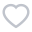256人关注
•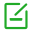卷面总分：100
•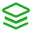试卷类型：模拟试题
•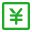测试费用： 免费
•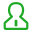关注人数：692
•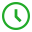作答时间：90分钟
•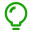答案：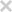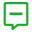试卷简介：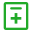试题类型：
• 单选题
• 共用备选答案单选题• 单选题
• 共用备选答案单选题
1

A.还原酶

B.脱氢酶

C.氧化酶

D.转氨酶

E.脱羧酶

2

A.絮状沉淀试验

B.环状沉淀反应

C.单向扩散试验

D.免疫透射比浊法

E.速率散射比浊法

3

A.＜2.8mmol/24h

B.＜3.8mmol/24h

C.＜4.8mmol/24h

D.＜5.0mmol/24h

E.＜5.8mmol/24h

4

A.0°～2°

B.2°～3°

C.3°～5°

D.5°～10°

E.5°～15°

5

A.是指单位标记物中结合于被标记物上的放射性强度

B.一般要求小于95%

C.常用的测定方法是利用三氯醋酸将待测样品中所有蛋白质沉淀

D.该参数是观察在洗脱期内标记物脱碘程度的重要指标

E.常用Ci/g、mCi/mg、Ci/mmol等单位表示

6

A.无痛性进行性淋巴结肿大

B.肝大

C.脾大

D.皮肤瘙痒

E.乏力

7

A.破坏蛋白质的一级结构

B.破坏蛋白质的二级结构

C.破坏蛋白质水化膜而改变溶解度

D.使蛋白质发生变性沉淀

E.使蛋白质等电点发生改变

8

A.AFP

B.CEA

C.CA125

D.CA153

E.PSA

9

A.对流免疫电泳

B.火箭免疫电泳

C.免疫电泳

D.免疫固定电泳

E.交叉免疫电泳

10

A.实践性、时代性、社会性

B.实践性、继承性、时代性

C.继承性、变革性、时代性

D.时代性、变革性、实践性

E.时代性、继承性、社会性

1

A.ROC曲线下面积(AUC)=0.1

B.ROC曲线下面积(AUC)=0.5

C.ROC曲线下面积(AUC)=0.8

D.ROC曲线下面积(AUC)=0.9

E.ROC曲线下面积(AUC)=1.0

2

A.ROC曲线下面积(AUC)=0.1

B.ROC曲线下面积(AUC)=0.5

C.ROC曲线下面积(AUC)=0.8

D.ROC曲线下面积(AUC)=0.9

E.ROC曲线下面积(AUC)=1.0

3

A.ROC曲线下面积(AUC)=0.1

B.ROC曲线下面积(AUC)=0.5

C.ROC曲线下面积(AUC)=0.8

D.ROC曲线下面积(AUC)=0.9

E.ROC曲线下面积(AUC)=1.0

4

A.ROC曲线下面积(AUC)=0.1

B.ROC曲线下面积(AUC)=0.5

C.ROC曲线下面积(AUC)=0.8

D.ROC曲线下面积(AUC)=0.9

E.ROC曲线下面积(AUC)=1.0

5

A.ROC曲线下面积(AUC)=0.1

B.ROC曲线下面积(AUC)=0.5

C.ROC曲线下面积(AUC)=0.8

D.ROC曲线下面积(AUC)=0.9

E.ROC曲线下面积(AUC)=1.0

6

A.脆弱拟杆菌

B.产气肠杆菌

C.粘质沙雷菌

D.粪肠球菌

E.雷极变形杆菌

7

A.脆弱拟杆菌

B.产气肠杆菌

C.粘质沙雷菌

D.粪肠球菌

E.雷极变形杆菌

8

A.脆弱拟杆菌

B.产气肠杆菌

C.粘质沙雷菌

D.粪肠球菌

E.雷极变形杆菌

9

A.审核

B.认可

C.认证

D.合格评

E.评审

10

A.审核

B.认可

C.认证

D.合格评

E.评审